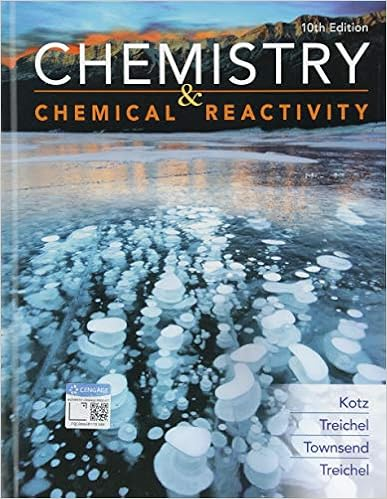# Avogadro&#39;s Law Figure 1 Helium Filled Balloons Why do...

• Lab Report
• 5
• 100% (3) 3 out of 3 people found this document helpful

This preview shows page 1 - 2 out of 5 pages.

##### We have textbook solutions for you!
The document you are viewing contains questions related to this textbook.The document you are viewing contains questions related to this textbook.
Chapter 2 / Exercise 30
Chemistry & Chemical Reactivity
Kotz/TreichelExpert Verified
Avogadro's Law Figure 1. Helium Filled Balloons Why do Helium Balloons Float in the Air? Have you ever wondered why helium filled balloons sold in stores ﬂoat in the air, but the ones you blow air into do not, even though they are the same size? The reason is that the same volume of helium weighs less than air. According to Avogadro's Law, balloons of similar volume contain similar number of moles of substance. However, helium is lighter than air because its molar mass is lower than oxygen's and nitrogen's, the two major components of air. Gases are different from liquids and solids in that they have neither a fixed volume nor a fixed shape. The behavior of gases depends on four variables: pressure ( P ), volume ( V ), temperature ( T ), and amount (number of moles, n ). There are three key laws that express the relationship between these variables: Boyle's , Charles's , and Avogadro's Law. These laws express the effect of one variable on another, while the other two variables remain constant. Avogadro's Law The Italian chemist and physicist, Amedeo Avogadro (1776 – 1856), established Avogadro's Law in 1811. This gas law states that equal volumes of gases at fixed temperature and pressure contain the same number of particles. This means that a sample of one gas under specific conditions of pressure and temperature will have the same number of moles as another gas, as long as the pressure and temperature are kept constant.
##### We have textbook solutions for you!
The document you are viewing contains questions related to this textbook.The document you are viewing contains questions related to this textbook.
Chapter 2 / Exercise 30
Chemistry & Chemical Reactivity
Kotz/TreichelExpert Verified
•••